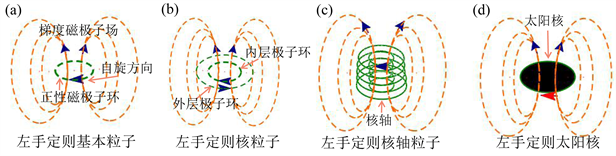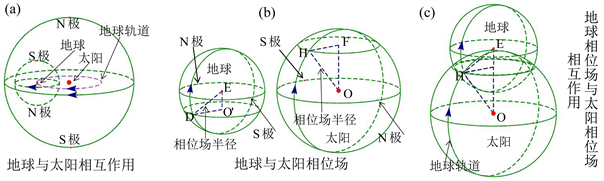#### 期刊菜单

Unified Phase Field Theory Based on the Interaction of Positive and Negative Magnetic Poles and NASA Astronomical Observation Data Analysis

Abstract: This article assumes that the elementary particle is a spin gradient magnetic pole field formed by the interaction of positive and negative magnetic poles. The galaxy nuclear, stellar nuclear, plane-tary nuclear and their spin gradient magnetic pole field are composed of the corresponding high- energy nuclear particle or nuclear axis particle. Dark matter refers to the galaxy nuclear particle, stellar nuclear particle, planetary nuclear particle and negative magnetic pole. Dark energy refers to the spin forces of the galaxy nuclear, stellar nuclear, planetary nuclear, and the expansion forces of high-density negative magnetic pole field. This article discusses that the gravitational forces, electromagnetic forces, strong forces and weak forces are all produced by the interaction of positive and negative magnetic poles. The binding forces of the positive magnetic poles ring coaxial spin of the nuclear particle is the strong forces, the decay forces of outer layer positive magnetic pole ring of the nuclear particle is the weak forces, the spin forces of the stellar (or planetary) gradient magnetic pole field on the corresponding orbit of the gravitational field is the electromagnetic forces (or kinetic energy), and the cohesive forces of the galaxy nucleus, stellar nucleus, and planetary nucleus to the magnetic pole of the gradient magnetic poles field (gravitational field) is the gravitational forces. This article attempts to discuss the unified phase field theory based on the interaction of positive and negative magnetic poles, and uses the curvature tensor equation of unified phase field to explains the unification of the gravitational force, electromagnetic force, strong force and weak force in the universe, and is verified by the astronomical observation data of the interactions be-tween the solar system and the Milky Way, the interactions between the planets and the solar system, the planetary perihelion precession. The NASA astronomical observation data is completely consistent with the calculated data of the phase field curvature tensor equation. Differential geometric variables are covariant with physical variables. The Lagrangian function of Einstein’s mass-energy equation, the Lagrangian function of the Schrodinger particle differential motion wave function based on the theory of relativity, the Lagrangian density of the Young-Mills gauge field equation, and the planets phase difference momentum-energy tensor of the curvature tensor equation is completely consistent in the interactions between planets and sun. These results prove that the unified phase field theory is in line with the physical reality of the universe.

1. 引言

1915年，爱因斯坦(A. Einstein)提出了可以揭示物质与四维时空之间联系的广义相对论 ，将其应用到宇宙学，得到一个初步的静态宇宙解 ，开启了现代宇宙学的大门。一个多世纪以来，许多科学家用“大爆炸理论”、“核合成理论”、“黑洞理论”等，解释宇宙现象，广泛开展天文科学观测，从不同角度对宇宙天体运动进行了深入探索。

1922年至1927年，弗里德曼(A. Friedmann)   和勒梅特(G. Lemaître) ，依据宇宙是均匀的、各向同性的这一假设，给出弗里德曼–勒梅特–罗伯逊–沃尔克(FLRW)度规，解出广义相对论引力场方程，认为宇宙正在膨胀。勒梅特还认为宇宙可能是由一个独特的“原子”产生的 。

1929年，哈勃(E. Hubble)通过对河外星系的观测，发现星系之间正在远离，认为宇宙正在膨胀 。1948年，伽莫夫(G. Gamow)、阿尔菲(R.A. Alpher)和贝特(H. Bethe)提出核合成理论，认为各种元素可能起源于宇宙的膨胀和温度的降低 。同年，阿尔菲(R.A. Alpher)和赫尔曼(R.C. Herman)预测宇宙中应该存在宇宙微波背景(CMB) 。1965年，彭齐亚斯(A. Penzias)和威尔逊(R. Wilson)在贝尔实验室发现温度大约为3K的CMB ，认为这是大爆炸理论的有力证据。

1990年，宇宙背景探测卫星COBE (Cosmic Background Explorer)初步测量的CMB可以很好地用2.735 ± 0.06 K的黑体辐射来拟合 。1992年，COBE探测到了CMB的各向异性，数量级大约为10−5 。2001年和2009年，威尔金斯微波各向异性探测器WMAD (Wilkinson Microwave Anisotropic Detector)和普朗克宇宙学探测器Planck (Planck Cosmological Detector)相继升空，其精度大大提高，从而可以进一步测量CMB的各项异性，并研究宇宙的各种组份。Planck卫星2018年的数据表明当前宇宙主要由大约4.9%的普通物质、大约26%的暗物质以及大约68%的可以提供负引力的暗能量组成 。

1993年，Phillips等人发现Ia型超新星可以作为标准烛光来确定距离  。1998年，索尔·珀尔马特(S. Perlmutter)和布赖恩·保罗·施密特(B.P. Schmidt)，以及亚当·里斯(Adam Guy Riess)通过对Ia型超新星的观测数据分析，得到宇宙在加速膨胀的结论  。

1933年，兹威基(F. Zwicky)用位力定理分析后发座星系团(Coma Cluster)中星系的速度弥散，发现后发座的平均密度应远大于可见物质的平均密度，即存在大量的暗物质   。1936年，史密斯(S. Smith)研究室女座星系团(Virgo Cluster)的质量，发现星系的平均质量比哈勃(E. Hubble)估计的质量大的多 。2015年，美国的激光干涉引力波天文台(Laser Interferometer Gravitational-Wave Observatory, LIGO)首次探测到了双黑洞并合释放出的引力波GWF150914 。

2. 基于正负磁极子相互作用的粒子自旋梯度磁极子场假设Figure 1. The interaction of the positive and negative magnetic poles of elementary particles and its gradient magnetic poles field. (a) The positive magnetic pole ring of the elementary particle drives the negative gradient magnetic pole field to spin in the external field, forming an energy field (or electromagnetic field) that conforms to the left-hand rule or the right-hand rule. (b) A nuclear particle is a composite particle composed of a pole ring of a large mass elementary particle nested in a pole ring of a small mass elementary particle, and the spin direction obeys the spin direction of the inner pole ring. For example, a neutron is formed by the pole ring of proton is nested in the pole ring of electrons. (c) The nuclear axis particle is formed by the coaxial superposition of the pole ring of the nuclear particle, and the spin direction obeys the spin direction of the inner pole ring, such as the atomic nuclear axis particle. (d) The galaxy nucleus, stellar nucleus, and planetary nucleus are the corresponding condensed bodies of nuclear axis particles. The galaxy nucleus and planetary nucleus conform to the right-hand rule, the stellar nucleus conform to the left-hand rule

2.1. 基本粒子自旋梯度磁极子场假设

${m}_{m}=m/\sqrt{1-\left({v}^{2}/{c}^{2}\right)}-m$ (1)

$v=k/\sqrt{{\lambda }_{m}}$${\lambda }_{m}={\left(k/v\right)}^{2}$ (2)

$E=r\frac{1}{2}\left(m+{m}_{m}\right)v={m}_{m}{c}^{2}$ (3)

$F=\sqrt{G\left({m}_{1}+{m}_{1m}\right)\left({m}_{2}+{m}_{2m}\right)/{r}^{2}}$ (4)

$M=e{\int }_{i=1}^{i=\alpha }\left(\left(m+{m}_{m}\right)/e\right)\left({\left(i+\delta \right)}^{3}-{i}^{3}\right)\text{d}V=\left(m+{m}_{m}\right){\int }_{i=1}^{i=\alpha }\left({\left(i+\delta \right)}^{3}-{i}^{3}\right)\text{d}V$ (5)

2.2. 核粒子与核轴粒子及其梯度磁极子场的自旋

2.3. 星系核及其梯度磁极子场与外场的相互作用

2.4. 恒星核及其梯度磁极子场与外场的相互作用

2.5. 行星核及其梯度磁极子场与外场的相互作用

3. 暗物质与暗能量及其运动形式

4. 基于星系、恒星、行星梯度磁极子场相互作用的统一相位场论

4.1. 统一相位场论Figure 2. Earth, Sun spin phase fields and their interactions. (a) The spin of the solar nucleus drives the spin of their entire gradient magnetic pole field, thereby driving the earth to move in orbit. (b) The wavelength of the earth motion is equal to the wavelength of the energy density of its orbit, and both the earth and the sun have corresponding spin phase fields and radius. (c) The opposite side of the inner triangle (DEO') (Figure 2(b)) in the earth phase field coincides with the opposite side of the inner triangle (HOF) (Figure 2(b)) in the solar phase field and to form an isosceles triangle (HOE) (Figure 2(c)) in the interaction between the earth spin phase field and the sun spin phase field

${r}_{\phi }=\alpha {\lambda }_{m}/2\pi$ (6)

${r}_{\phi }={r}_{F}/T$ (7)

${\phi }_{\mu \nu }={g}^{\mu \nu }\left(\partial {q}_{\mu \nu }/q\right)$ (8)

4.2. 统一相位场曲率张量方程

${\varphi }_{\mu \nu }={R}_{\mu \nu }-\frac{1}{2}{\phi }_{\mu \nu }R=\frac{r}{{f}_{\mu \nu }{r}_{F}^{2}}{T}_{\mu \nu }=\frac{r}{{p}_{\mu \nu }{r}_{F}^{2}}{M}_{\mu \nu }$ (9)

4.3. 粒子相位场运动的拉格朗日量

${L}_{E}=E\frac{i\partial }{\partial r}={\left(m/\sqrt{1-{\left({v}_{t1}/c\right)}^{2}}-m\right)}^{2}-{\left(m/\sqrt{1-{\left({v}_{t2}/c\right)}^{2}}-m\right)}^{2}$ (10)

${L}_{\psi }=E\psi \frac{i\partial }{\partial r}=i\beta {h}_{m}\frac{\partial }{\partial t}\psi \left(r,t\right)=\left[-\frac{{\beta }^{2}{h}_{m}^{2}}{2m+{m}_{m}}{\nabla }^{2}+V\left(r,t\right)\right]\psi \left(r,t\right)$ (11)

$\lambda$ 是粒子运动波长， $\alpha$ 是精细结构常数。 ${h}_{m}$ 是基于粒子运动波长的静止质量常数，

${h}_{m}=5.45025577174353×{10}^{-65}\text{ }\text{ }\text{kg}\cdot {\text{m}}^{3}$$-\frac{{\beta }^{2}{h}_{m}^{2}}{2m+{m}_{m}}{\nabla }^{2}$ 是粒子动能算符( $\stackrel{^}{T}$ )， ${\nabla }^{2}$ 是拉普拉斯算符。 $V\left(r,t\right)$

$\begin{array}{c}{L}_{F}={F}_{E}\frac{i\partial }{\partial r}=\frac{1}{4}{F}_{\mu \nu }\cdot {F}^{\mu \nu }-\frac{1}{4}{F}_{\nu \mu }\cdot {F}^{\nu \mu }\\ =\frac{1}{4}{F}_{\mu \nu }\cdot {F}^{\mu \nu }-\frac{1}{2}{F}_{\nu \mu }\cdot \left({\partial }^{\mu }{A}^{\nu }-{\partial }^{\nu }{A}^{\mu }+g{A}^{\mu }×{A}^{\nu }\right)\end{array}$ (12)

${L}_{T}=F\frac{i\partial }{\partial r}=\sqrt{G\left({m}_{1}+{m}_{1m}\right)\left({m}_{2}+{m}_{2m}\right)/{r}_{F1}^{2}}-\sqrt{G\left({m}_{1}+{m}_{1m}\right)\left({m}_{2}+{m}_{2m}\right)/{r}_{F2}^{2}}$ (13)

5. 天文观测数据检验

5.1. 太阳系与银河系相互作用数据检验Table 1. Data of the interaction between the solar system and the Milky WayTable 2. Data of the interaction between the solar system and the Milky Way

5.2. 行星与太阳相互作用数据检验Table 3. Data of the interaction between planets and the sunTable 4. Data of the interaction between planets and the sun

5.3. 太阳系行星轨道进动数据检验Table 5. Precession data of the solar system planetary orbits in the century

$\epsilon =2\varphi -2\pi =\frac{3GW}{{c}^{2}}\pi \left(\frac{1}{{r}_{1}}+\frac{1}{{r}_{2}}\right)$，或 $\epsilon =24{\pi }^{3}\frac{{a}^{2}}{{T}^{2}{c}^{2}\left(1-{e}^{2}\right)}$ (14)Table 6. Precession data of the solar system planetary orbits in the centuryTable 7. Precession data of the solar system planetary orbits in the century

${M}_{\mu \nu }$ 作为拉格朗日密度， ${M}_{\mu \nu }$ 是行星最近一个世纪减少的能量(或动能)，可分别由式(10)、式(11)、和式(12)计算。 ${T}_{\mu \nu }$ 是行星最近一个世纪减少的引力，可由式(13)计算。Table 8. Precession data of the solar system planetary orbits in the century

6. 结论